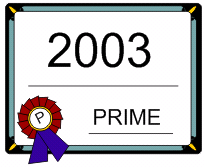PRIMES is in P. Where in P?

User Rating:/ 1
PoorBest

..

In 2002-2003 Agrawal, Kayal, and Saxena in a great breakthrough "PRIMES is in P"   ( Wiki EN ) proved that the PRIMES function (prime number test)  is O(log ^8 n), in other words polynomial of degree 8 of the number of digits (log n) of a given number n.

CongratulationsBTW, where in P is its real position? Any idea?

Notice that the Prime Number Test

PRIMES(n) = "n is/not a prime number"

does not solve the Integer Factorization Problem

IFP(x) = "x is/not pq, for integers p, q>1" .

For complexity of many problems check great pages ( Wiki EN, Wiki ENEN, EN, EN ).